# Closed-Loop Control of Variable Optical Attenuators with Logarithmic Analog Processing

Achieving tight channel spacing in dense wavelength-division-multiplexing (DWDM) networks calls for precise control of spectral emissions and power. This requires continuous monitoring and adjustment of network elements—such as transmission laser sources, optical add-drops, optical amplifiers, and variable optical attenuators (VOAs). These last elements are typically used to adjust power levels across the DWDM spectrum in order to minimize crosstalk and maintain a desired signal-to-noise ratio.

For instance, a VOA can be cascaded with an erbium-doped fiber amplifier (EDFA) to help equalize the amplifier’s non-uniform gain-versus-wavelength profile, improving linearity and enhancing control of the overall system. Recursive measurement-and-control algorithms can be used to provide quick and accurate dynamic closed-loop control, which ensures repeatability and minimizes production calibration and trimming. Logarithmic-amplifier front ends condition the wide-range input signal, thus allowing lower-resolution, lower-cost signal-processing components to be used downstream.

### Classic Mixed-Signal Solutions

Classic solutions have combined linear transimpedance amplifiers (TIAs) and high resolution signal processing to measure and control the absorbance of the VOA. This, at first, seems like an attractive solution due to the low cost of the TIA front end. The TIA is linear, however, so calculating the decibel (logarithmic) attenuation across the VOA requires post-processing of the measured signal. Performed digitally, this requires floating-point processors to cope with the division and exponentiation processes involved in the calculation. Alternatively, integer-based processing can be performed—using exhaustive look-up tables that were generated during production calibration. Both approaches typically require analog-to-digital converters with at least 14-bit resolution, and moderately high processor speeds, to minimize measurement latency resulting from the inherent processing overhead. The cost advantage sought in selecting the linear TIA front end is often swamped by the cost of the higher priced converters and processors needed to acquire the measurement signal and compute the attenuation. Additional cost (and production delay) is incurred if lengthy look-up tables need to be generated during production testing.Figure 1. VOA adaptive control loop. Input and output optical taps are used to measure the attenuation of the VOA. The measured signal is compared to a desired setpoint level using an error integrator, implemented in software. The difference signal is used to control the attenuation of the VOA, yielding a closed-loop design.

### Adaptive Control of a Variable Optical Attenuator

Figure 1 illustrates this classic solution applied around an adaptively controlled VOA. The amplified signal is low-pass filtered to help reduce measurement noise. The filtered signal is then digitized and the absorbance of the attenuator is computed.

If linear amplification is used to convert the measured photocurrents to proportional voltages, it will be necessary to calculate the ratio of the measured signals, followed by inverse exponentiation, and multiplication to compute the VOA’s actual absorbance.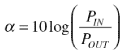(1)

If the responsivities and transimpedance gain of the detector front ends are equal, then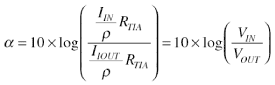(2)

where RTIA is the transresistance in ohms

ρ is the responsivity of the photodiode in A/W

In reality the front end transresistances will not be equal, so additional calibration and correction is required. In a digital solution, in order to provide acceptable precision in calculation, the signal needs to be digitized using analog-to-digital converters (ADCs) with sufficient resolution to preserve a predetermined level of accuracy. The design can operate as a closed loop only as long as the input signal is large enough for the detected signals to be above the noise floor. Variable transresistance may be used to help extend the range of closed-loop operation. When the measured signal levels fall below an acceptable signal level for accurate detection, the VOA must indicate the lack of signal power and operate open-loop, and is no longer able to fulfill the accuracy requirement.

### To the Rescue—Translinear Log-Amp Circuits

Published in 1953, the Ebers-Moll equations predicted the inherent logarithmic relationship between the base-emitter voltage (VBE) and the collector current (IC) of a bipolar transistor.1 When fabricated on a high quality analog process, this relationship is remarkably accurate over an IC range of up to 9 decades. Significant advances occurred, starting with the exploitation of the logarithmic properties in the 1960s.2 A significant landmark was Barrie Gilbert’s description of the power(s) inherent in their generalized translinear properties.3 Over the years, the logarithmic and related properties of bipolar junctions have been used to create a variety of wide-range linear and nonlinear devices, including precision multipliers and dividers, rms-to-dc converters,4 and modulators. They have made possible the compact, wide-range, precision analog solution to be described.

Briefly, over a range of about 9 decades, a BJT exhibits a naturally logarithmic relationship between its collector current and its base-to-emitter voltage,(3)

where IS is the transport saturation current, of the order of 10–16 A, k/q is the ratio of Boltzmann’s constant to the charge on an electron (1/11,605 V/K), and T is the absolute temperature in kelvins. The thermal voltage, kT/q, is thus simply proportional to absolute temperature (PTAT), about 25.85 mV at 300 K. Unfortunately, the current IS is poorly defined, differing significantly between separate devices; it is also strongly dependent on temperature, varying by a factor of roughly 109 between –35°C and +85°C. A single BJT alone would be a highly impractical log-amp because of these strong temperature dependencies. To make use of BJTs for accurate logarithmic transformations, the temperature dependencies must be nullified.

The difference between the base-emitter voltages of a pair of BJTs, one operating at the photodiode current, IPD, and the second operating at a reference current, IREF, can be expressed as(4)

If the two transistors are closely matched such that they exhibit nearly identical saturation currents, then Equation 4 can be written as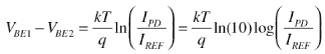(5)

At an ambient temperature of 300 K, the above equation evaluates to(6)

The poorly defined and temperature dependent saturation current, IS, which appears in Equation 1, has now been eliminated. To eliminate the temperature variation of kT/q, this difference voltage is applied to an analog divider with a denominator that is directly proportional to absolute temperature. The final output voltage is now essentially temperature independent and can be expressed as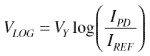(7)

The logarithmic slope, VY, is expressed in volts per decade. Typical translinear log-amps such as the ADL5306 and ADL5310 are scaled to provide a nominal 200 mV/decade slope. The logarithmic slope can be increased or decreased with the addition of a simple fixed-gain amplifier or voltage attenuator network.

When light falls on a photodiode, the resulting photocurrent is directly proportional to the incident optical power in watts. On a decibel scale, the decibels of optical power are proportional to the logarithm of the photocurrent, scaled by the responsivity of the particular photodiode. The decibel power of any photodiode can be written as(8)

If the reference current in the denominator of Equation 7 is known, the absolute power incident on the photodiode can be calculated from the VLOG voltage.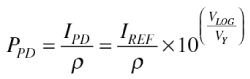(9)

In terms of decibels of optical power(10)

Using Equation 10 we can solve for the log-voltage in terms of optical power in dB.(11)

where(12)

This provides a linear-in-dB transfer function, allowing a simple straight-line equation to describe the relationship of absolute optical power to the logarithmic output voltage. In practice, the actual reference current value and responsivity of the photodiode will be found through a simple two-point calibration process. By measuring the output voltage for two known values of optical power, the slope and intercept of the straight-line equation can be determined. Then simple subtraction and multiplication can be used to assess the optical power in decibels without the need for the exponentiation process or the exhaustive look-up table that would be required if a linear TIA front end were applied.

If the numerator current is derived from the photocurrent, IPD, of an input tapped detector, and the denominator current, IREF, is the resulting photocurrent after the input signal has passed through an absorptive element, such as a VOA, Equation 7 can then be used to derive the attenuation in terms of the log-voltage.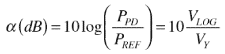(13)

A similar expression could be derived for an optical amplifier design, where it is desirable to compute the gain in dB across the amplifier. As before, a simple two-point calibration is used to describe the straight-line equation relating the output voltage to the EDFA gain setting. In this manner, translinear log-amps provide the capability to measure absolute power, optical absorbance, and gain using a simple straight-line approximation.

Recent advances in monolithic integrated-circuit design now allow for the manufacture of translinear logarithmic amplifiers that provide wide-dynamic-range signal compression virtually free from the temperature dependencies inherent in a discrete implementation. The first complete monolithic translinear logarithmic amplifier (log-amp), the AD8304, designed by Barrie Gilbert, was introduced by Analog Devices in January 2002. Since the introduction of the AD8304, logarithmic devices have become available from other semiconductor manufacturers.

### Application to a VOA

In a digitally variable optical attenuator (DVOA) application, input and output optical taps are used to measure the absolute optical power at the input and output ports. The power measurements can then be processed to compute the absorbance of the DVOA for a particular attenuation setting. Using closed-loop techniques, the attenuation can be dynamically controlled to maintain a desired level of attenuation based on the input and output power measurements. This mode of operation is called automatic attenuation control, or AAC. An alternate mode, automatic power control (APC), is necessary when the output power of the DVOA is required to remain at a constant level regardless of input optical power variations (as long as the input level exceeds the desired output level by the minimum insertion loss of the VOA).

In either mode of operation, the loop is typically implemented in the form of a mixed-signal solution, where a digital ADL5310 dual translinear logarithmic amplifier, which is capable of interfacing two independent photodiodes for measurement of the absolute power on two separate optical supervisory channels. This device allows for absolute measurement of the output optical signal, while simultaneously providing a measure of the attenuation.

### Automatic Attenuation Control and Automatic Power Control

Two buffered outputs can be obtained by utilizing the ADL5310’s on-chip op amps. The circuit configuration of Figure 2 makes available a measurement of the absolute power incident on photodiode PD1, as well as a measure of absorbance observed between the two ports. Test results using an uncalibrated VOA are provided in Figure 3. The outputs provide the desired linear-in-dB transfer functions needed for closed-loop analog control. Automatic attenuation and power control can be achieved by applying the appropriate output to a separate error integrator; its output drives the control voltage of the VOA.

The solution in Figure 2 assumes that the individual logarithmic-slopes of each channel are identical. In reality the channel-to-channel slope mismatch could be as high as 5%. This will result in a residual error as the overall power levels change. Over a 5-decade power range, the residual error could be as much as 2.5 dB. This can be acceptable in some situations—where fast all-analog closed-loop control is necessary, and accuracy can be compromised in order to allow a simple hardware solution. In a solution where mixed-signal techniques can provide fast enough response, the residual error could be predicted and minimized by monitoring the absolute power on one detector and using a look-up table for error correction.Figure 2. Hardware implementation of VOA control using a logarithmic front end. The ADL5310 is configured to provide an absolute power measurement of the optical signal power incident on PD1, while the difference voltage reveals the absorbance across the VOA. The wide dynamic range allows the loop to remain locked over a broad range of input signals.

The test results in Figure 3 are subject to the inaccuracies of the VOA used in the lab. The measurement was repeated with calibrated current sources to better assess the accuracy of the design. Figure 4 illustrates the full dynamic range capabilities and log-conformance of the circuit in Figure 2. The accuracy is better than 0.1 dB over a 5-decade range.Figure 3. Transfer functions for the two outputs. Channel 1 provides the absolute output power, while Channel 2 provides the relative attenuation between the two channels.Figure 4. Transfer functions for the two outputs.Figure 5. Log-conformance of the transfer functions.

An alternative solution is offered in Figure 6, where a current mirror is used to feed an opposite-polarity replica of the cathode photocurrent of PD2 into Channel 2 of the ADL5310. The current mirror shown is a modified Wilson mirror. While other current-mirror circuits would also work, this modified Wilson mirror provides fairly constant performance over temperature. To minimize the effects of temperature gradients and beta mismatch, it is essential to use matched-pair transistors when designing the current mirror.

The circuit of Figure 6 is no longer subject to the logarithmic-slope mismatch issues inherent in the previous solution. Individual channel slope and intercept characteristics can now be calibrated independently. The accuracy was verified using a pair of calibrated current sources in order to eliminate any error due to the integral nonlinearity of the VOA. The performance of the circuit depicted in Figure 6 is shown in Figures 7 and 8. Transfer functions and error plots are provided for several power levels. The accuracy is better than 0.1 dB over a 5-decade range. The dynamic range is slightly reduced for strong IIN input currents. This is due to the limited available swing of the VLOG pin; it can be improved through careful selection of input and output optical-tap coupling ratios.

### Conclusion

Translinear log-amps simplify VOA absorbance measurements by providing a linear-in-dB relationship between the input photocurrent and resulting output voltage. The division and exponentiation processes that would be required with a linear solution are eliminated when using logarithmic signal processing. There are several ways of using translinear log-amps to provide absorbance and absolute power measurements for AAC and APC control loops. A simple difference amplifier arrangement, using on-chip operational amplifiers, provides a compact solution, but it may require additional error correction if logarithmic slope mismatch is unacceptable. A slightly more complicated solution involves using a modified Wilson current-mirror. The current-mirror approach is essentially immune to channel-to-channel mismatch and is capable of providing more than 5 decades of measurement and control range.

### 参考資料

1 This outstandingly important facet of the bipolar junction transistor (BJT) is a feature of the deeply fundamental physics that govern minority-carrier conduction in a junction device. An early paper on the subject is: “Large-signal behavior of junction transistors,” Ebers, I., and J. Moll, Proceedings of the IRE, December 1954, pp. 1761-1772.

2 “Multiplication and logarithmic conversion by operational-amplifier-transistor circuits,” Paterson, W., Review of Scientific Instruments, 34-12, December 1963.

3 “Translinear circuits: a proposed classification,” Gilbert, B., Electronics Letters, 11- 1, 1975, pp. 14-16.

4 Nonlinear Circuits Handbook, Engineering Staff of Analog Devices, Inc., Sheingold, D., ed. Norwood, MA: Analog Devices, Inc. (1974). (out of print)